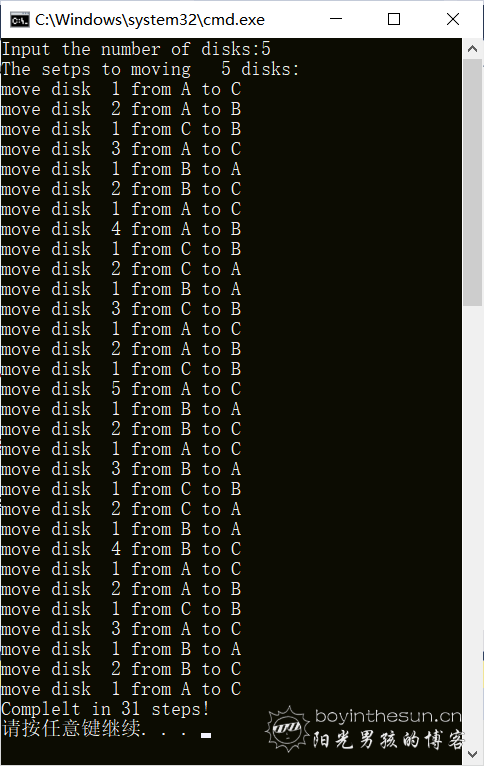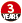# 基于C语言用递归算法实现汉诺塔解法的计算

2020-04-05 12:16 92 查看

```#include<stdio.h>

int steps = 0;

//三根柱A(用0表示)，B(用1表示)，C(用2表示)，起始A，目标C

void print(int num, int get, int put) {
steps++;
printf("move disk%3d from %c to %c\n", num, get == 0 ? 'A' : get == 1 ? 'B' : 'C', put == 0 ? 'A' : put == 1 ? 'B' : 'C');
}
void mv(int num,int a,int b,int c) {
if (num == 1)
print(1, a, c);
else {
mv(num - 1, a, c, b);
print(num, a, c);
mv(num - 1, b, a, c);
}
}
int main() {
int n;
printf("Input the number of disks:");
scanf("%d", &n);
printf("The setps to moving %3d disks:\n",n);
mv(n, 0, 1, 2);
printf("Complelt in %d steps!\n",steps);
return 0;
}```## 交流讨论等具体内容请访问我的博客

• 点赞
• 收藏
• 分享
• 文章举报BoyInTheSun 发布了16 篇原创文章 · 获赞 0 · 访问量 1332 私信 关注